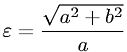Equations > Geometry > Curves and Shapes > Eccentricity of a Hyperbola

### Eccentricity of a HyperbolaLatex Code:

MathML Code:

 $ϵ=\frac\sqrt{{a}^{2}+{b}^{2}}a$

MathType 5.0: